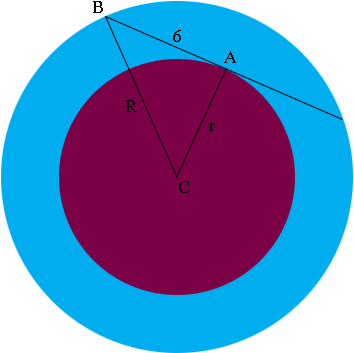SEARCH HOMEMath Central Quandaries & QueriesQuestion from maribie, a student: two circles are concentric. the tangent to the inner circle forms a chord of 12cm in the larger circle. find the area of the "ring" between the two circles?Hi Maribile,

Suppose that the radius larger circle is $R$ and the radius of the smaller circle is $r.$ Write an expression for the area of the ring between the two circles.

Next look at a diagram of the two circles.$AB$ is perpindcular to $CA$, do you see why? What does Pythagoras say about the triangle $ABC?$

PennyMath Central is supported by the University of Regina and The Pacific Institute for the Mathematical Sciences.